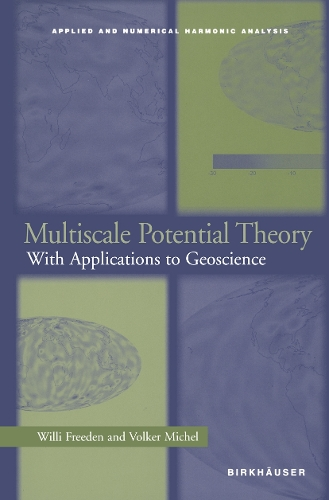•Multiscale Potential Theory: With Applications to Geoscience - Applied and Numerical Harmonic Analysis (Paperback)

(author), (author)
£59.99
Paperback 510 Pages / Published: 12/10/2011
• We can order this

Usually dispatched within 3 weeks

This self-contained text/reference provides a basic foundation for practitioners, researchers, and students interested in any of the diverse areas of multiscale (geo)potential theory. New mathematical methods are developed enabling the gravitational potential of a planetary body to be modeled using a continuous flow of observations from land or satellite devices. Harmonic wavelets methods are introduced, as well as fast computational schemes and various numerical test examples. Presented are multiscale approaches for numerous geoscientific problems, including geoidal determination, magnetic field reconstruction, deformation analysis, and density variation modelling

With exercises at the end of each chapter, the book may be used as a textbook for graduate-level courses in geomathematics, applied mathematics, and geophysics. The work is also an up-to-date reference text for geoscientists, applied mathematicians, and engineers.

Publisher: Springer-Verlag New York Inc.
ISBN: 9781461273950
Number of pages: 510
Weight: 797 g
Dimensions: 235 x 155 x 27 mm
Edition: Softcover reprint of the original 1st ed. 200

MEDIA REVIEWS

"The book is devoted to well-posed and ill-posed boundary-value problems arising in geoscience, elasticity, gravimetry and other areas, including satellite problems. New mathematical methods and fast computational schemes based on harmonic analysis and wavelet transforms are developed.... The book may be used for graduate-level courses in geomathematics, applied mathematics, and geophysics. It is also an up-to-date reference text for geoscientists, applied mathematicians, and engineers." -Zentralblatt MATH

"Potential theory is a classical area in mathematics which for over 200 years has attracted and still attracts attention. Famous mathematicians have contributed. At the beginning of the 19th century it was Laplace, Poisson, Gauss, Green, both F. and C. Neumann, Helmholtz, Dirichlet and others. In the last century axiomatic, fine, probabilistic, discrete, and nonlinear potential theory arose.

The present book is written for applications in geodesy and geophysics and is hence devoted to classical potential theory with particular attention to wavelet approximation.... Each chapter is concluded with exercises which have solution hints at the end of the book.... The book is a self-contained and unique presentation of multiscale potential theory, interesting for applied mathematicians, geophysicists, etc. and proper even for students." -Mathematical Reviews